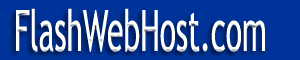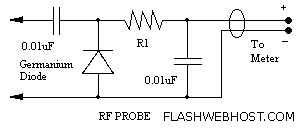FlashWebHost.com  |   Resources  |   Ham Radio Circuits  |   Ham Radio  |   SSB Transceiver  |

# Designing RF Probes

An RF probe is used to directly measure the level of RF voltage present at a particular point and is one of the most useful test instrument in the hands of the home brewer. It is normally used with a digital multi meter to indicate the voltage level as dc voltage which is equivalent to the RMS value of the RF voltage being measured.

However, the level of RF voltage being measured provides useful information only when the probe has been designed for use with a specific multi meter. The design of the RF probe is a function of the DC input resistance of the meter we intend to use with it. If a new meter with a different input resistance is used with the probe the reading will be inaccurate.

Look at the figure below which shows the construction of the RF probe. The rectified DC voltage at the cathode of the diode is at about the peak level of the RF voltage at the tip. The value of the resistor R1 is so chosen that when this resistor is connected in parallel with the input resistance of the digital multi meter, the peak value is about 1.414 times the RMS voltage. R1 has to drop this excess voltage so the meter indication is accurate. If we know the input resistance of the meter, we can calculate the value of R1 as follows. Usually, digital multi meters have an input resistance of 11 meg ohms. In this example we shall take the input resistance of the meter as 10 meg ohms which will make calculation easier to understand.

10,000,000 X 1.414 = 14,140,000
R1 = 14,140,000 - 10,000,000 = 4,140,000 Ohms = 4.14 Meg Ohms
4.7 meg ohms is the value chosen in all circuits since digital multimeters have input resistance of 11 meg ohms.
Circuit By RAMASWAMI VU2PRI.